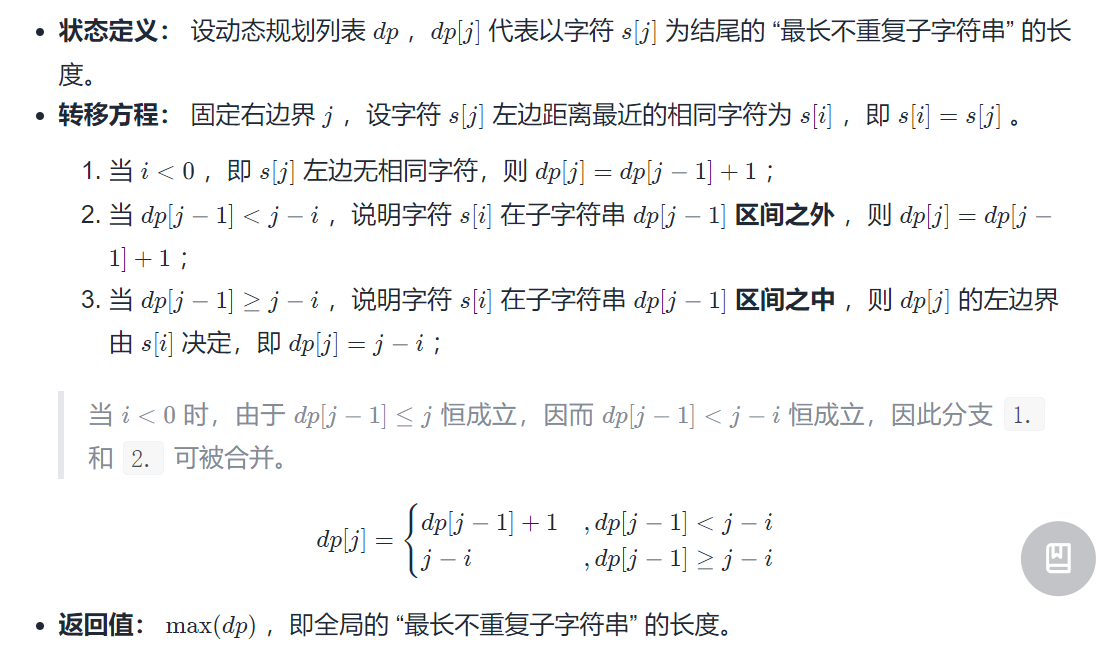## 题目

• 请从字符串中找出一个最长的不包含重复字符的子字符串，计算该最长子字符串的长度。

• 示例1

• 示例2

• 示例3

## 代码

• 双指针+哈希表
class Solution {
// 滑动窗口，用set维护一个不重复的窗口
public int lengthOfLongestSubstring(String s) {
int res = 0;
Set<Character> set = new HashSet<>();
for(int l = 0, r = 0; r < s.length(); r++) {
char c = s.charAt(r);
while(set.contains(c)) {
set.remove(s.charAt(l++));
}
res = Math.max(res, r - l + 1);
}

return res;
}
}


• dp+哈希表+优化
public int lengthOfLongestSubstring(String s) {
Map<Character, Integer> dic = new HashMap<>();
int res = 0, tmp = 0;
for(int j = 0; j < s.length(); j++) {
// getOrDefault(key, default)
// 代表当哈希表包含键 key 时返回对应 value ，不包含时返回默认值 default
int i = dic.getOrDefault(s.charAt(j), -1); // 获取索引 i
dic.put(s.charAt(j), j); // 更新哈希表
tmp = tmp < j - i ? tmp + 1 : j - i; // dp[j - 1] -> dp[j]
res = Math.max(res, tmp); // max(dp[j - 1], dp[j])
}
return res;
}


代码分析详解 代码是相当的巧妙、本文题目zui-chang-bu-han-zhong-fu-zi-fu-de-zi-zi-fu-chuan-lcof